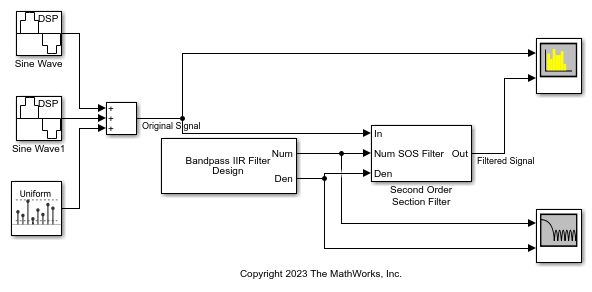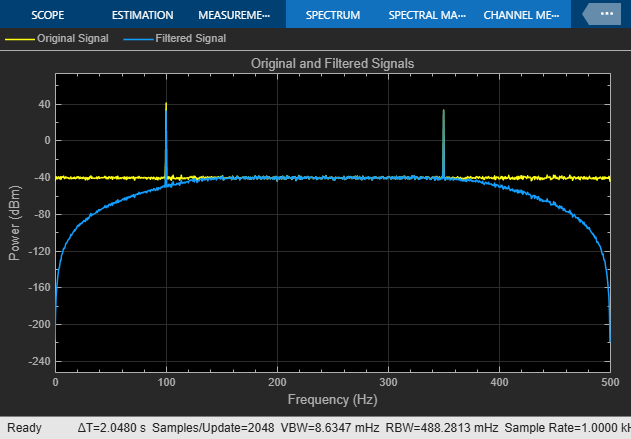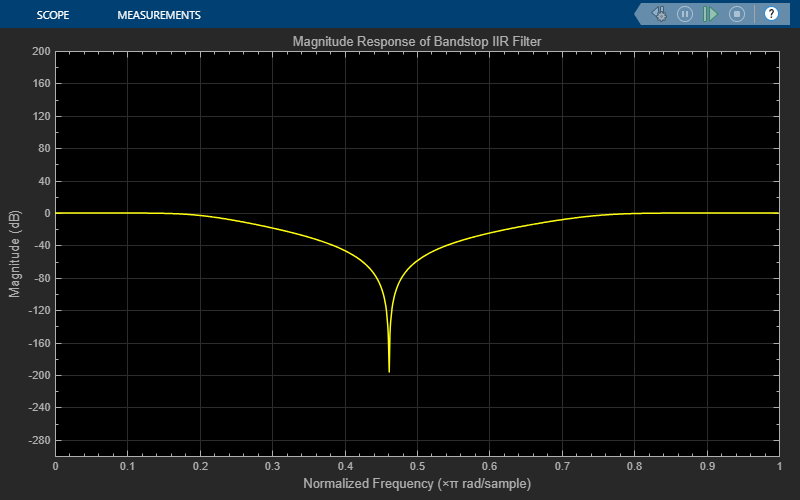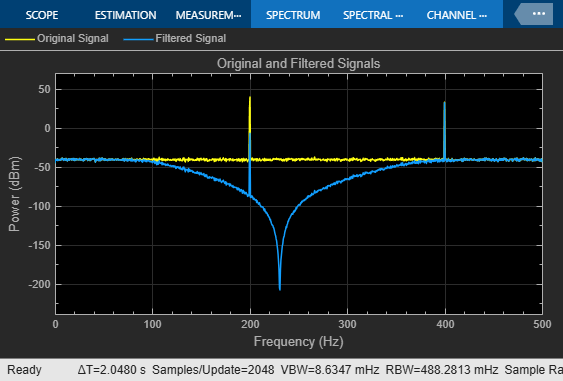# Second-Order Section Filter

Implement cascade of second-order section filters

Since R2023b

•Libraries:
DSP System Toolbox / Filtering / Filter Implementations

## Description

The Second-Order Section Filter block implements a cascade of second-order section (SOS) filters in Simulink®. You can specify the numerator and denominator coefficients of the filter in the block parameters dialog box or through input ports.

## Examples

expand all

Filter a noisy sinusoidal signal using the Second-Order Section Filter block. Obtain the numerator and denominator coefficients of the SOS filter using the Tunable Bandpass IIR Filter block.

Tune the frequency specifications of the SOS filter during simulation.

Open and Run Model

Open the secondordersectionfilter model by clicking the Open Model button.

The input signal in the model is a sum of two sine waves with the frequencies of 100 Hz and 350 Hz. The sample rate is 1000 Hz and the number of samples in each frame is 1024. The Random Source block adds zero-mean white Gaussian noise with a variance of 1e-4 to the sum of the sine waves.

The Tunable Bandpass IIR Filter block designs a sixth-order bandpass IIR filter with the first and second 3-dB cutoff frequencies of 0.25rad/sample and 0.75rad/sample, respectively. The block generates coefficients as a cascade of second-order sections. Visualize the frequency response of the filter using the Filter Visualizer.

Run the model.

The Second-Order Section Filter block filters the noisy sinusoidal signal. Visualize the original sinusoidal signal and the filtered signal using the Spectrum Analyzer.Tune Frequency Specifications of SOS Filter

During simulation, you can tune the frequency specifications of the SOS filter by tuning the frequency parameters in the Tunable Bandpass IIR Filter block. The frequency response of the SOS filter updates accordingly.

Change the second 3-dB cutoff frequency to 0.4rad/sample in the Tunable Bandpass IIR Filter block. The second tone of the sinusoidal signal gets attenuated as it no longer falls in the passband region of the filter.Filter a noisy sinusoidal signal using the Second-Order Section Filter block. Obtain the numerator and denominator coefficients of the SOS filter using the Tunable Bandstop IIR Filter block.

Tune the frequency specifications of the SOS filter during simulation.

Open and Run Model

Open the secondordersection_bandstopfilter model by clicking the Open Model button.

The input signal in the model is a sum of two sine waves with the frequencies of 200 Hz and 400 Hz. The sample rate is 1000 Hz and the number of samples in each frame is 1024. The Random Source block adds zero-mean white Gaussian noise with a variance of 1e-4 to the sum of the sine waves.

The Tunable Bandstop IIR Filter block designs a sixth-order bandstop IIR filter with the first and second 3-dB cutoff frequencies of 0.2rad/sample and 0.75rad/sample, respectively. The block generates coefficients as a cascade of second-order sections. Visualize the frequency response of the filter using Filter Visualizer.

Run the model.

The Second-Order Section Filter block filters the noisy sinusoidal signal. Visualize the original sinusoidal signal and the filtered signal using the Spectrum Analyzer. The first tone is attenuated as it falls in the stopband region of the filter while the second tone remains unaffected as it falls in the passband region of the filter.Tune Frequency Specification of SOS Filter

During simulation, you can tune the frequency specifications of the SOS filter by tuning the frequency parameters in the Tunable Bandstop IIR Filter block. The frequency response of the SOS filter updates accordingly.

Change the first 3-dB cutoff frequency to 0.5rad/sample in the Tunable Bandstop IIR Filter block. The first tone of the sinusoidal signal now falls in the passband region and is therefore unattenuated.## Ports

### Input

expand all

Specify the input signal as a vector or a matrix. The input can be a variable-size signal, that is, the frame size of the signal can change during simulation but the number of channels cannot.

This port is unnamed until you set the Coefficient source parameter to Input ports.

Data Types: single | double
Complex Number Support: Yes

Specify the numerator coefficients of the SOS filter as a P-by-3 matrix, where P is the number of filter sections. For more details on this input port, see Numerator.

During simulation, you cannot change the size of the coefficients matrix but you can change the coefficient values.

The size and complexity of the Num and Den inputs must be the same.

#### Dependencies

To enable this port, set the Coefficient source parameter to Input ports.

Data Types: single | double
Complex Number Support: Yes

Specify the denominator coefficients of the SOS filter as a P-by-3 matrix or a P-by-2 matrix, where P is the number of filter sections. For more details on this input port, see Denominator.

When you specify a P-by-3 matrix, the block always assumes the leading denominator coefficient to be 1. If you specify any other value in the first column, the block ignores this value and treats it as 1. If you specify a P-by-2 matrix, the block prepends each row of the matrix with a value of 1.

During simulation, you cannot change the size of the coefficients matrix but you can change the coefficient values.

The size and complexity of the Num and Den inputs must be the same.

#### Dependencies

To enable this port, set the Coefficient source parameter to Input ports.

Data Types: single | double
Complex Number Support: Yes

Specify the scale values that the block applies before and after each section of the SOS filter as a scalar or a vector with P + 1 elements, where P is the number of filter sections.

If you input a scalar, the block applies this gain value before the first filter section and sets the gain values before all the other sections to 1. If you specify a vector of P + 1 values, the block applies each value to a separate section of the filter.

Tunable: Yes

#### Dependencies

To enable this port, set Coefficient source to Input ports and select the Specify scale values parameter.

Data Types: single | double

### Output

expand all

Filtered output, returned as a vector or a matrix. The output has the same size and data type as the input. The output signal is complex if the input signal, numerator coefficients, or the denominator coefficients is complex.

This port is unnamed until you set the Coefficient source parameter to Input ports.

Data Types: single | double
Complex Number Support: Yes

## Parameters

expand all

To edit block parameters interactively, use the Property Inspector. From the Simulink Toolstrip, on the Simulation tab, in the Prepare gallery, select .

Specify the filter structure as one of these:

• Direct form I

• Direct form I transposed

• Direct form II

• Direct form II transposed

Specify the filter coefficient source as one of these:

• Dialog parameters –– Set the filter coefficients and scale values through the Numerator, Denominator, and Scale Values parameters in the block dialog box.

• Input ports –– Set the filter coefficients and the scale values through the Num, Den, and g input ports.

Specify the numerator coefficients b of the SOS filter as a P-by-3 matrix, where P is the number of filter sections.

$b=\left[\begin{array}{ccc}{b}_{01}& {b}_{11}& {b}_{21}\\ {b}_{02}& {b}_{12}& {b}_{22}\\ ⋮& ⋮& ⋮\\ {b}_{0P}& {b}_{1P}& {b}_{2P}\end{array}\right]$

This equation represents the SOS filter in the transfer function form.

$H\left(z\right)=\prod _{k=1}^{P}{H}_{k}\left(z\right)=\prod _{k=1}^{P}\frac{{b}_{0k}+{b}_{1k}{z}^{-1}+{b}_{2k}{z}^{-2}}{{a}_{0k}+{a}_{1k}{z}^{-1}+{a}_{2k}{z}^{-2}},$

where

• a is the denominator coefficients matrix. For more details on how to specify this matrix, see Denominator.

• k is the row index.

You cannot change the size of this parameter during simulation, but you can change its value.

Tunable: Yes

#### Dependencies

To enable this parameter, set Coefficient source to Dialog parameters.

Data Types: single | double
Complex Number Support: Yes

Specify the denominator coefficients a of the SOS filter as a P-by-3 matrix or a P-by-2 matrix, where P is the number of filter sections.

$a=\left[\begin{array}{ccc}{a}_{01}& {a}_{11}& {a}_{21}\\ {a}_{02}& {a}_{12}& {a}_{22}\\ ⋮& ⋮& ⋮\\ {a}_{0P}& {a}_{1P}& {a}_{2P}\end{array}\right]$

The block algorithm assumes that the value of the leading coefficients is always 1. If the denominator is of size P-by-2, the block algorithm prepends a column of 1s to the denominator to change its size to P-by-3. If the denominator is of size P-by-3 and the elements in the first column do not equal 1, the algorithm ignores the values in the first column and sets them to 1.

This equation represents the SOS filter in the transfer function form.

$H\left(z\right)=\prod _{k=1}^{P}{H}_{k}\left(z\right)=\prod _{k=1}^{P}\frac{{b}_{0k}+{b}_{1k}{z}^{-1}+{b}_{2k}{z}^{-2}}{{a}_{0k}+{a}_{1k}{z}^{-1}+{a}_{2k}{z}^{-2}},$

where

• b is the numerator coefficients matrix. For more details on how to specify this matrix, see Numerator.

• k is the row index.

You cannot change the size of this parameter during simulation, but you can change its value.

Tunable: Yes

#### Dependencies

To enable this parameter, set Coefficient source to Dialog parameters.

Data Types: single | double
Complex Number Support: Yes

Specify if the filter has scale values for each section. When you select this parameter, you can specify the scale values using the Scale Values parameter in the block dialog box or through the g input port.

Specify the scale values that the block applies before and after each section of the SOS filter as a scalar or a vector with P + 1 elements, where P is the number of filter sections.

If you set this property to a scalar, the block applies this gain value before the first filter section and sets the gain values before all the other sections to 1. If you set this property to a vector of P + 1 values, the block applies each value to a separate section of the filter.

Tunable: Yes

#### Dependencies

To enable this parameter, set Coefficient source to Dialog parameters and select the Specify scale values parameter.

Data Types: single | double

Specify the input processing method as one of these:

• Columns as channels (frame based) –– The block treats each column of the input as a separate channel.

• Elements as channels (sample based) –– The block treats each element of the input as a separate channel.

Open the Filter Visualization tool and display the magnitude response of the SOS filter. The response is based on the parameters you select in the block dialog box. To update the magnitude response, modify the parameters in the dialog box and click .## Block Characteristics

 Data Types double | single Direct Feedthrough no Multidimensional Signals no Variable-Size Signals yes Zero-Crossing Detection no